## 1. 时间序列定义及分类

$\left \{ x_{t},t=-2,-1,0,1,2,...,T \right \}$

1. 时间序列按照有没有趋势分为：

• 平稳序列：不存在趋势的序列，各观察值在固定水平上波动。
• 非平稳序列：包含趋势，季节性或周期性的序列，可包含一种或多种。
2. 通常时间序列模型会按照周期的长短分成四个影响因子：

• 趋势（Trend）：在长时期呈现出的单调上升或者下降的趋势，如长期经济增长，某新兴行业的规模。
• 季节性（seasonality）：这个是指在一年内重复出现的周期波动，并在每一年同一时期都出现相似的趋势，如春夏秋冬，上下半年，淡旺季。
• 周期性（cyclicity）：通常是由经济环境变化引起的，而且不同于趋势变动的涨落交替波动。周期性变动时长经常超过一年。
• 随机性（Irregular）：由偶然因素影响的不规则变动，这种影响因子在时间序列中是不可预估的，通常为噪声。

影响因子在time series analysis工具包中可以用下列python语句来分析：

result = sm.tsa.seasonal_decompose(data, freq=365)
复制代码

## 2. 时间序列模型

### 2.1 线性模型

#### 2.1.1 自回归模型（AR）

Auto Regressive模型是线性时间序列模型中最简单的的模型，他是通过前面部分的数据与后面部分的数据之间的相关关系来建立回归方程的。由线性回归发展而来。当一个时间序列 $x_1$, $x_2$, $x_3$, ... $x_n$ ，则其p阶AR模型 $AR(p)$ 的数学表达式为：

$x_{t}=\phi _{1}x_{t-1}+\phi _{2}x_{t-2}+...+\phi _{p}x_{t-p}+u_{t}$

• AR模型对偏自相关函数截尾，对自相关函数拖尾（截尾表示从某阶开始均为（接近）0，拖尾指并不存在某阶突然跳变0而是逐渐衰减到0，而时间序列自相关衡量得失序列自身在不同时刻随机变量的相关性，偏自相关测提出了两时刻之间其他随机变量的干扰，是更纯粹的相关。）。

• 在AR模型建模的过程中，确定p阶数的问题叫做定阶，一般常用偏自相关函数和AIC准测。具体做法为用PACF画图来判断其截尾性，以选取最好的阶数p。

• 模型参数估计方法可用最小二乘法，最大似然估计。

• AR模型在金融模型中主要是对金融序列过去进行建模。

#### 2.1.2 滑动平均模型（MA）

Moving Average也是线性时间序列模型中的一种，与AR模型不同的地方在于，它并非是时间历史序列值的线性组合，而是历史白噪声的线性组合来影响当前时刻点的预测值。他的原理是历史白噪声是通过影响历史时序值，从而间接影响到当前时刻点的预测值。如果一段白噪声序列 $u_1$, $u_2$, $u_3$,... $u_n$,则q阶MA模型 $MA(q)$的数学表达式为：

$x_{t}=u_{t}+\theta _{1}u_{t-1}+\theta _{2}u_{t-2}+...+\theta _{q}u_{t-q}$

• MA的定阶理论在自相关系数在q后截尾，所以查看ACF图最后一个显著不等于0的位置可以暂定为MA的阶。实际可以用AIC定阶。
• MA的参数估计可以用矩估计法，逆相关函数法，条件最大似然估计法和精确最大似然估计法。
• 在金融模型中MA通常用来描述冲击效应。

#### 2.1.3 自回归滑动平均模型（ARMA）

$x_{t}=\phi _{1}x_{t-1}+\phi _{2}x_{t-2}+...+\phi _{p}x_{t-p}+u_{t}+\theta _{1}u_{t-1}+\theta _{2}u_{t-2}+...+\theta _{q}u_{t-q}$

• ARMA的定阶通常是在一个范围内通过观察搜索最小AIC以及BIC的方式来选取阶数组合，可以用网格搜索的方法来求。

#### 2.1.4 差分自回归滑动平均模型（ARIMA）

ARMA模型可以处理平稳时间序列，但是对于非平稳时间序列，则需要引入差分来解决这个问题，所以ARIMA模型出现。其数学表达形式为：

$\left ( 1-\sum_{i=1}^{p}\phi _{i}L^{i} \right )\left ( 1-L \right )^{d}X_{t}=\left ( 1+\sum_{i=1}^{q}\theta _{i}L^{i} \right )\varepsilon _{t}$

$\Delta ^{d}x_{t}=\left ( 1-L \right )^{d}X_{t}$

$wt$ 等于：

$w_{t}=\Delta ^{d}x_{t}=\left ( 1-L \right )^{d}x_{t}$

$w_{t}=\phi _{1}w_{t-1}+\phi _{2}w_{t-2}+...+\phi _{p}w_{t-p}+\delta +u_{t}+\theta _{1}u_{t-1}+\theta _{2}u_{t-2}+...+\theta _{q}u_{t-q}$

ARIMA的开发流程为

1. 数据可视化，识别平稳性。
2. 对非平稳的时间序列数据做差分，得到平稳序列。
3. 建立合适的模型：
• 平稳化处理后，若PACF是截尾的，而ACF是拖尾的，则建立AR模型；
• 若PACF是拖尾的，而ACF是截尾的，则建立MA模型；
• 若PACF和ACF均是拖尾的，则序列适合ARMA模型；
4. 模型的阶数在确定之后，对ARMA模型进行参数估计，比较常用是最小二乘法进行参数估计。
5. 假设检验，判断（诊断）残差序列是否为白噪声序列。
6. 利用已通过检验的模型进行预测。

1. 用pandas读取和加载数据，并同时做好数据预处理，处理空值以及时间点缺失。
2. 使用可视化对数据进行探索，通过图表了解基本的统计和可能存在的季节性趋势。
3. 选择模型，规定网格搜寻的参数范围，以最低AIC得分穷举最佳参数组合。
4. 设置需要预测的时间段，然后用最优模型来做predict。

#### 2.1.5 季节性ARIMA（SARIMA）

SARIMA：因为时间序列中定义了季节性，而含有季节性差异的ARIMA模型。季节性差异和常规差异相似，但是可以通过从上一个季节中减去该值而不是减去连续项来实现。SARIMA的阶数组合为：

$\left ( p,q,d \right )\times \left ( P,Q,D,m \right )$

SARIMAX：而再SARIMA上再加入外生变量。

#### 2.1.6 模型评估

AIC：从预测角度衡量统计模型拟合优良性标准，AIC越小，模型越好，通常选择AIC最小的模型

$AIC=2k-2\ln \left ( L \right )=\frac{\left ( 2k-2L \right )}{n}$
• k是参数数量，k小意味着模型简洁；
• L是似然函数， $L=-\left ( \frac{n}{2} \right )\ln \left ( 2\pi \right )-\left ( \frac{n}{2} \right )\ln \left ( \frac{sse}{n} \right )-\frac{n}{2}$，sse是残差平方和。L大意味着模型精确；
• n是观测值数目；

BIC：贝叶斯信息准则，从拟合角度衡量模型对数据拟合的优良，同样也是BIC越小模型越好

$BIC=k\ln\left ( n \right )-2\ln \left ( L \right )$

HQ $BIC=k\ln\left ( \ln(n) \right )-2\ln \left ( L \right )$

### 2.2 非线性模型LSTM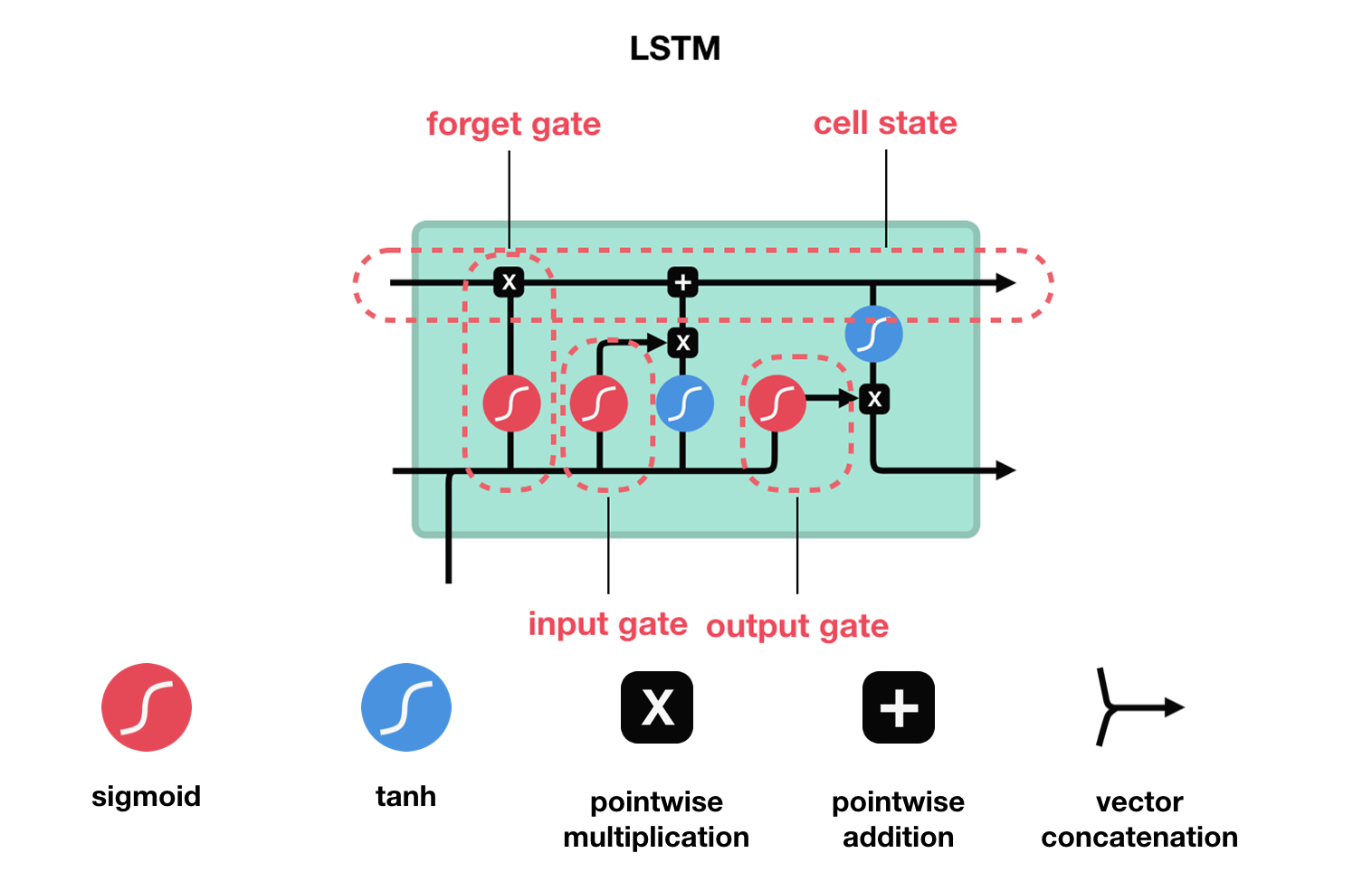#### 2.2.1 遗忘门（forget gate）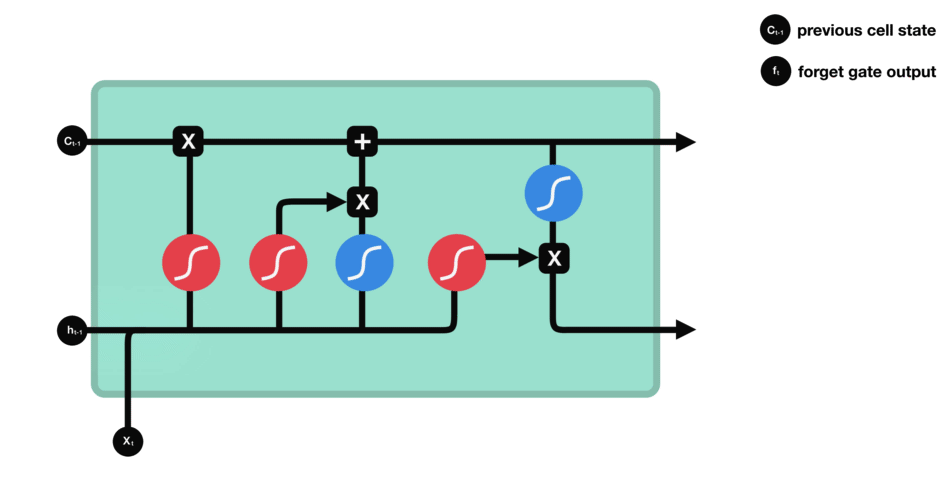#### 2.2.2 输入门（input gate）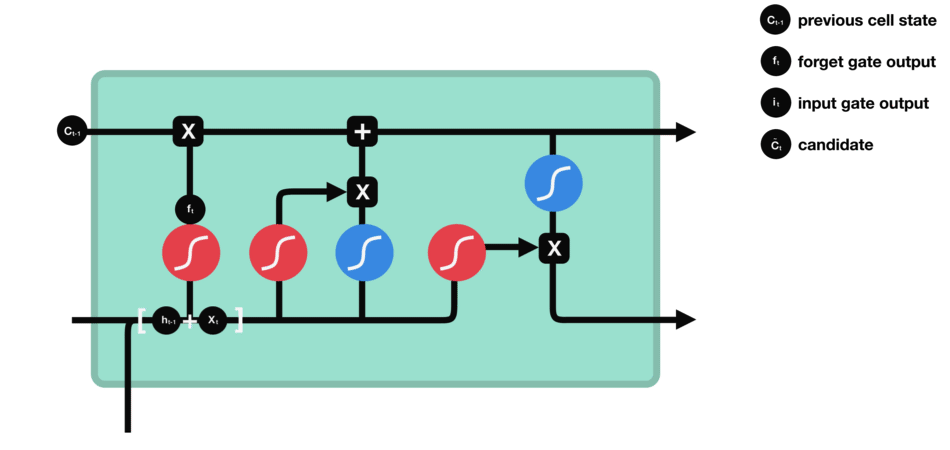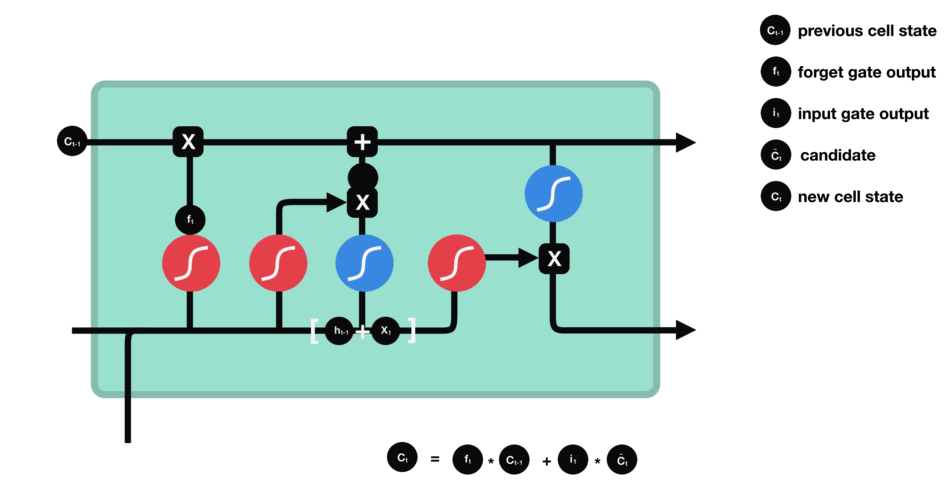#### 2.2.3 输出门（output gate）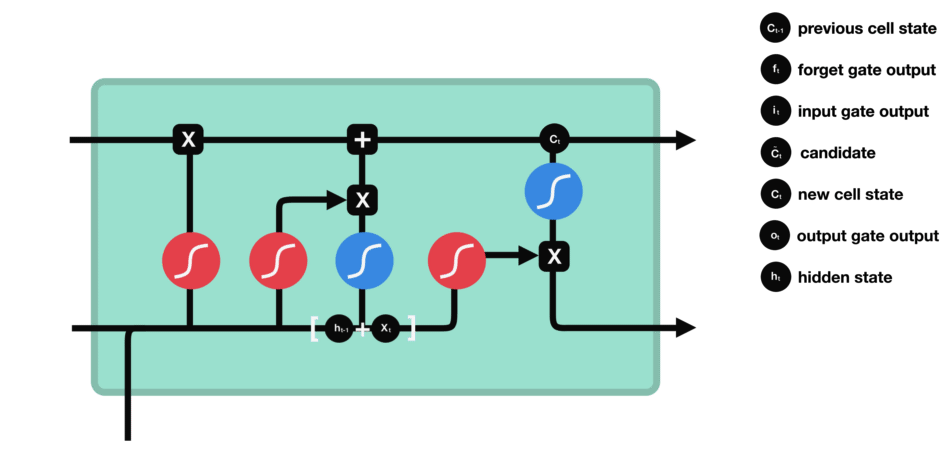#### 2.2.4 数学表示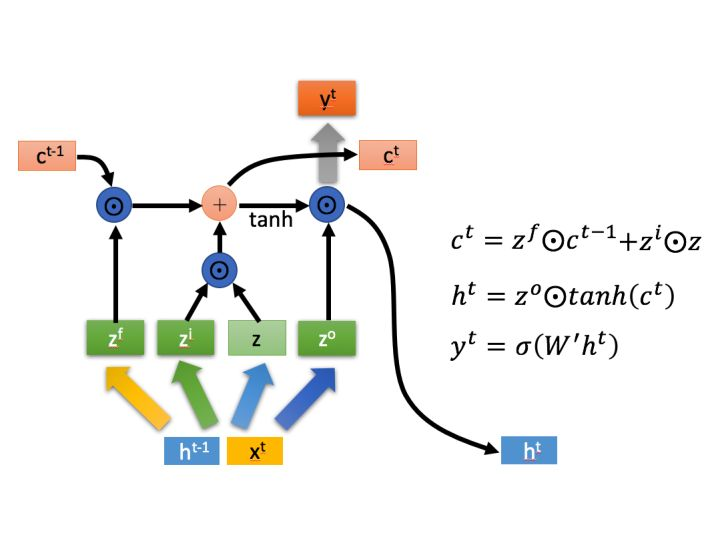$c^{t}=z^{f}\odot c^{t-1}+z^{i}\odot z$

$h^{t}=z^{o}\odot tanh(c^{t})$

$y^{t}=\sigma \left ( W{}'h^{t} \right )$

LSTM时序项目开发流程

1. 数据预处理，并将数据集变成滑动窗口形式的时序数据；
2. 搭建lstm模型（lstm层，dense层，优化器，损失函数）；
3. 拟合模型，最好设置定时保存模型，以获得最佳拟合模型；
4. 对未来时间数据进行预测。Python

493